阵列天线的赋形波束综合(二)---伍德沃德—劳森抽样法

2022-01-13 来源：微波射频网 作者：94巨蟹座少年 字号：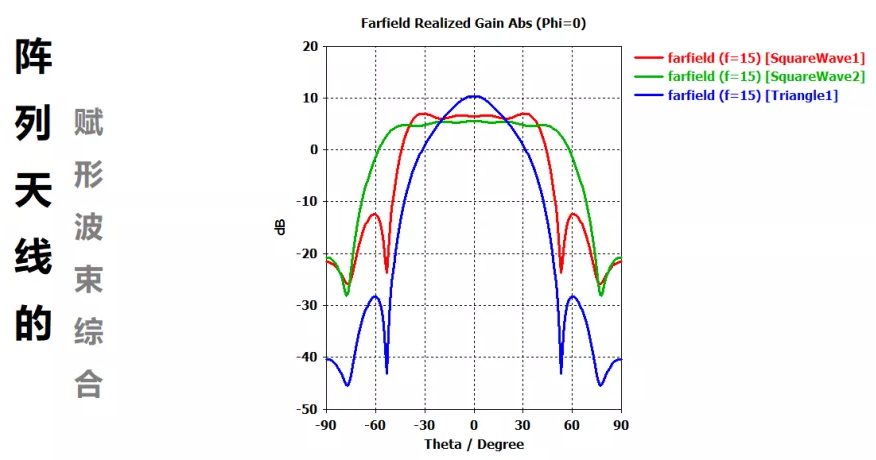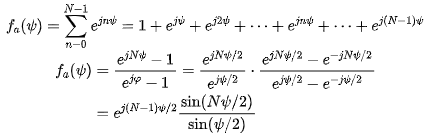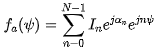1. P. M. Woodward, “A Method for Calculating the Field over a Plane Aperture Required to Produce a GivenPolar Diagram,” J. IEE, Vol. 93, pt. IIIA, pp. 1554–1558, 1946.

2. P. M. Woodward and J. D. Lawson, “The Theoretical Precision with Which an Arbitrary Radiation-Pattern May be Obtained from a Source of a Finite Size,” J. IEE, Vol. 95, pt. III, No. 37, pp. 363–370, September 1948.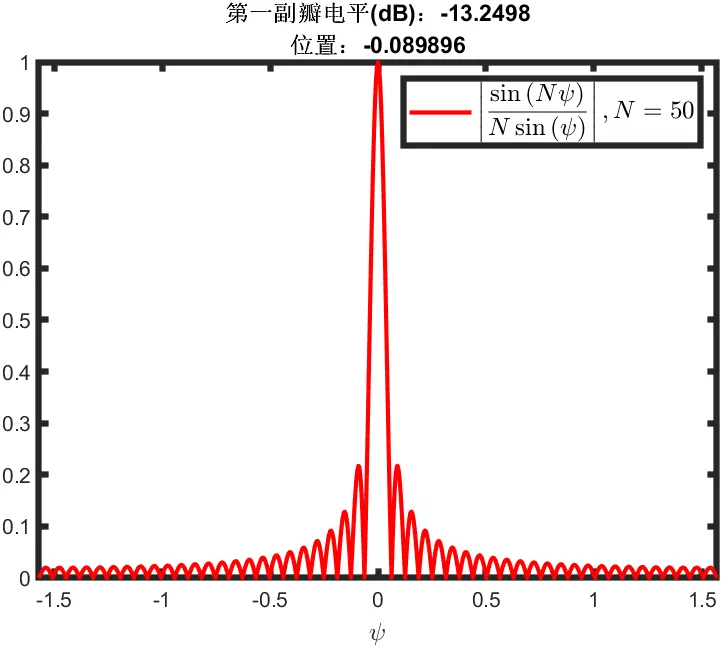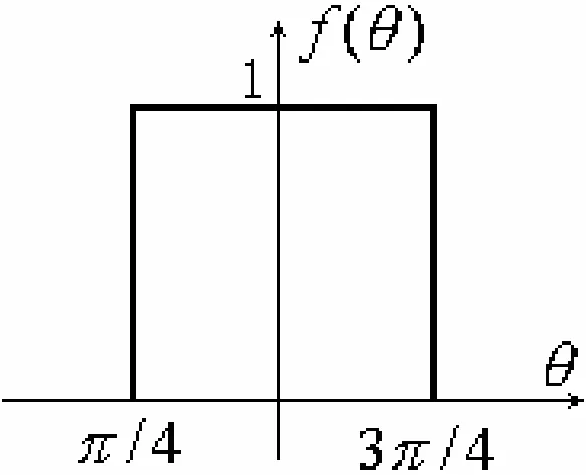%matlab code clear;clc;N=10;format long; color_array={'r','g','b','m','k'}; theta0_array=45:10:135;legend_str={}; h=figure;set(gcf,'Position',[100 100 800 600]); for num=1:length(theta0_array)     theta=0:0.1:180;theta0=theta0_array(num);     F=abs(sin(N*deg2rad(theta-theta0))./(N*sin(deg2rad(theta-theta0))));     plot(theta,F,color_array{mod(num,length(color_array))+1},'linewidth',2);     set(gca,'linewidth',3);     xlabel('\theta(°)','Fontsize',12);     ylabel('F(\theta)','Fontsize',12);     set(gca,'XTick',0:10:180);     set(gca,'YTick',0:0.1:1);     axis([0,180,0,1]);     legend_str{num}=strcat(num2str(theta0),'°');     hold on; end h=legend(legend_str); set(h,'FontName','Times New Roman','FontSize',12,'FontWeight','normal'); hold off;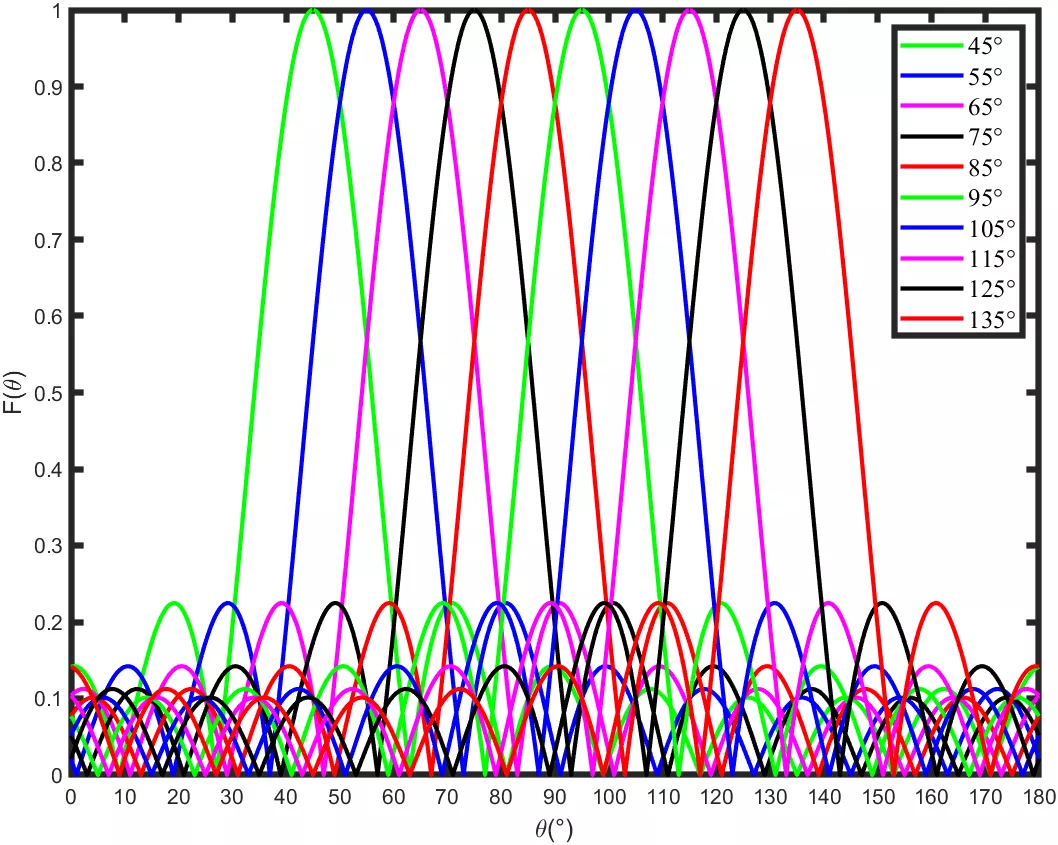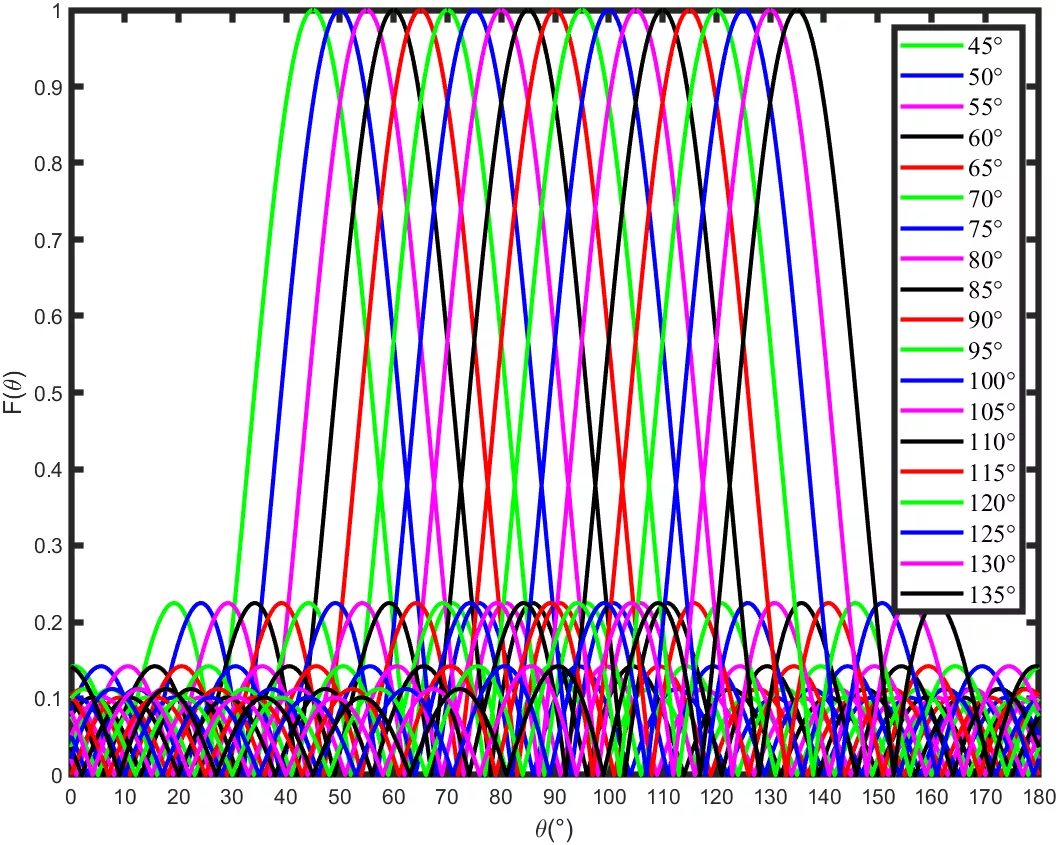%matlab code clear;clc;N=10;format long; theta0_array=45:5:135; h=figure;set(gcf,'Position',[100 100 800 600]); Ftotal=0; for num=1:length(theta0_array)     theta=0:0.1:180;theta0=theta0_array(num);     F=abs(sin(N*deg2rad(theta-theta0))./(N*sin(deg2rad(theta-theta0))));     Ftotal=Ftotal+F; end F_uniform=Ftotal/max(Ftotal); plot(theta,F_uniform,'r','linewidth',2); set(gca,'linewidth',3); xlabel('\theta(°)','Fontsize',12); ylabel('Ftoal(\theta)','Fontsize',12); set(gca,'XTick',0:10:180); set(gca,'YTick',0:0.1:1); axis([0,180,0,1]);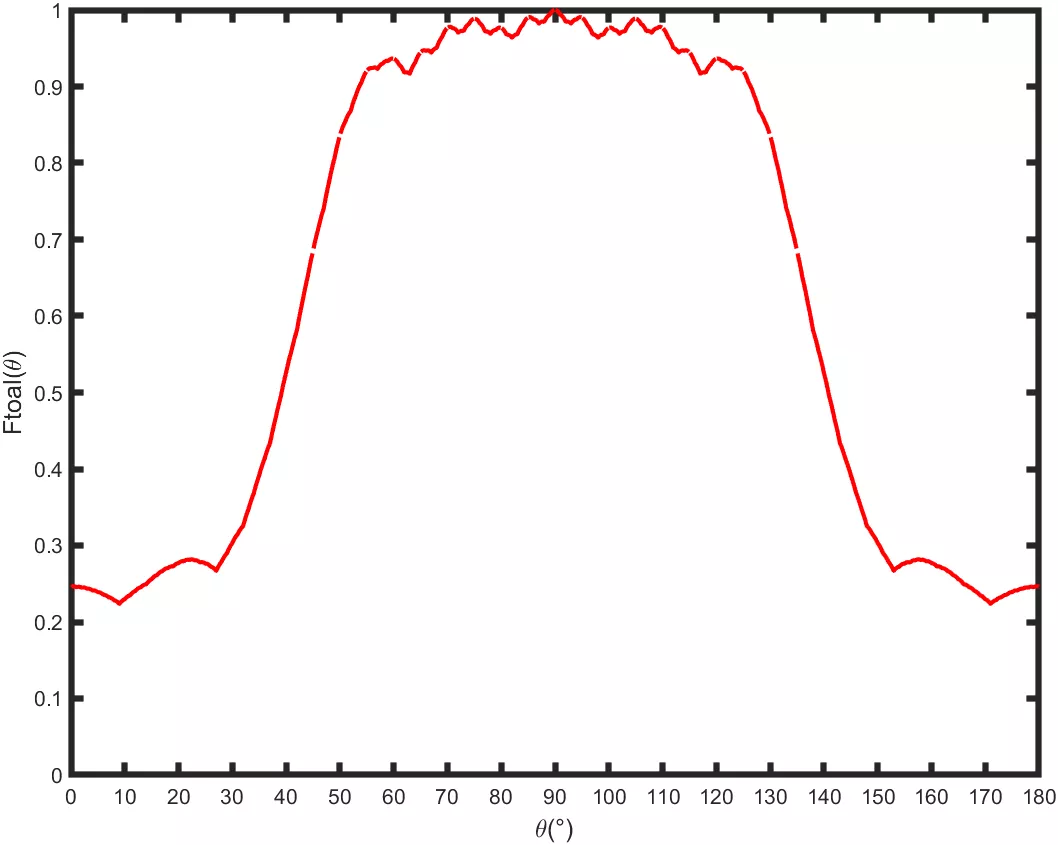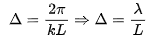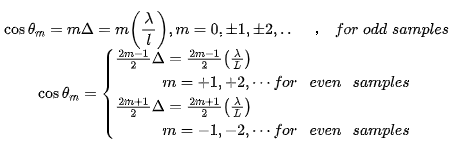clear;clc; lambda_d=0.2; for m=-5:1:5     theta_m=rad2deg(acos(m*lambda_d)); end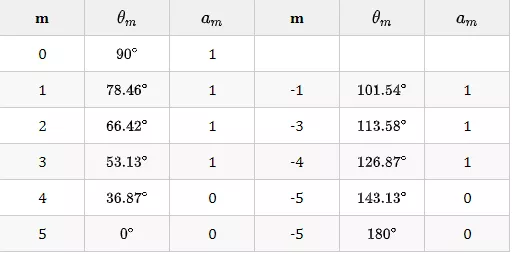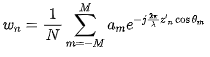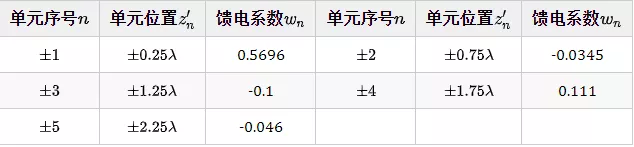CST实例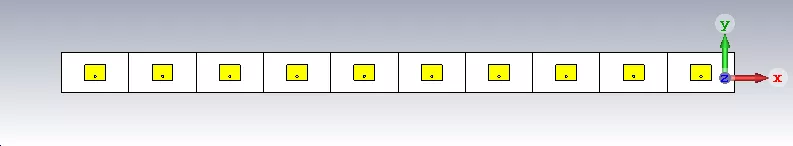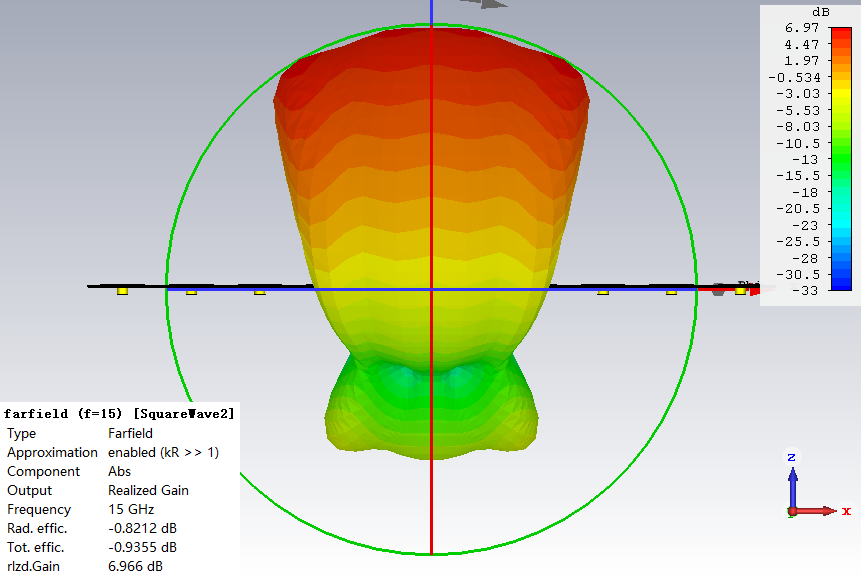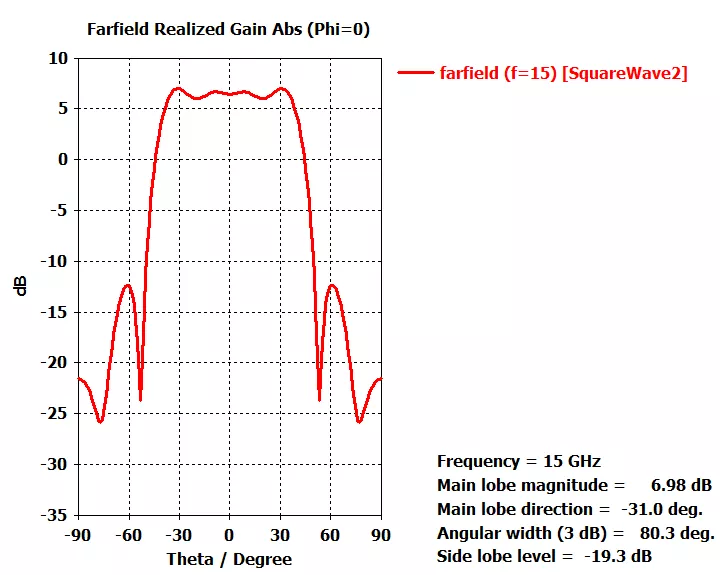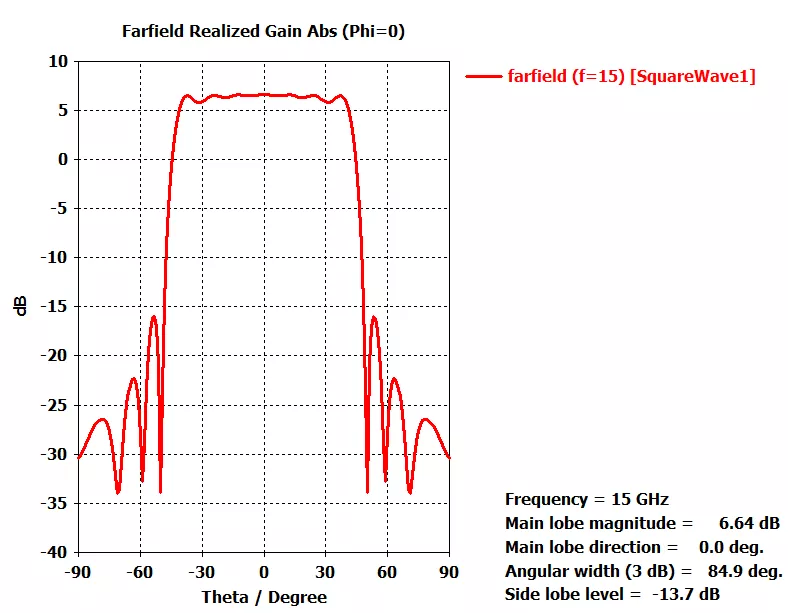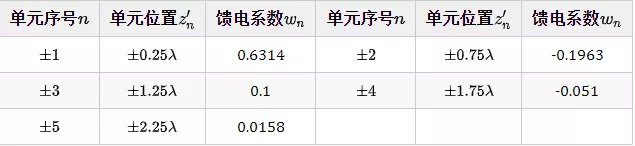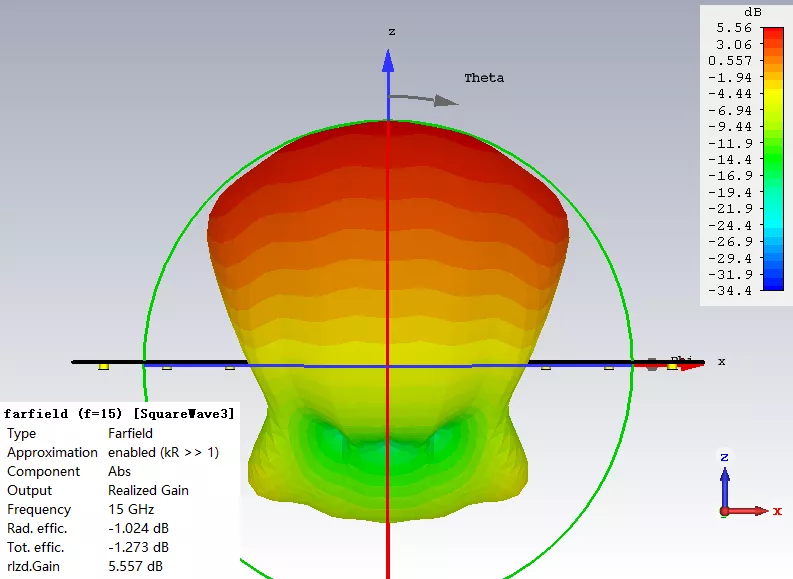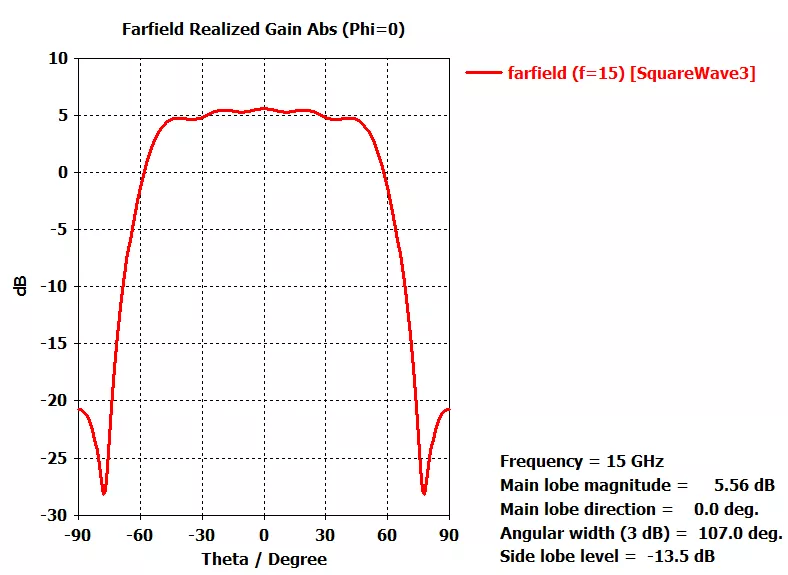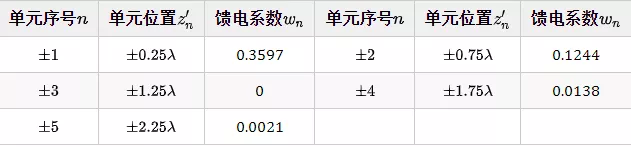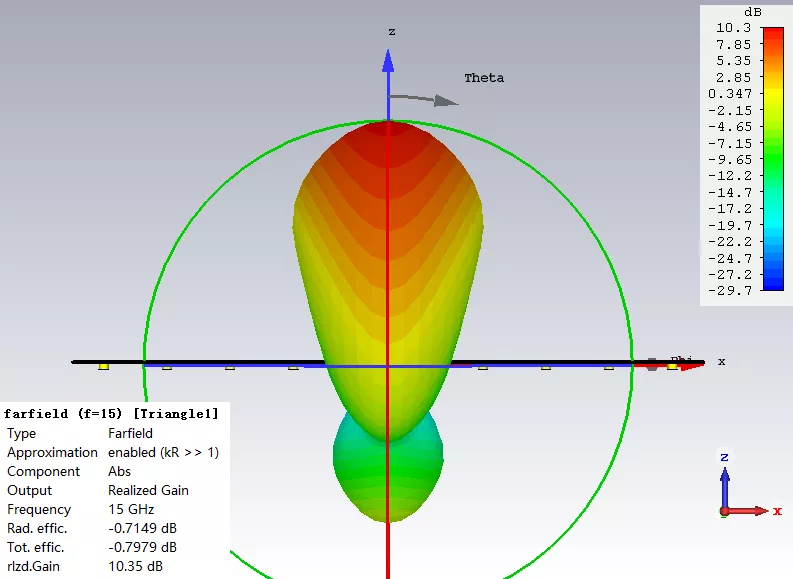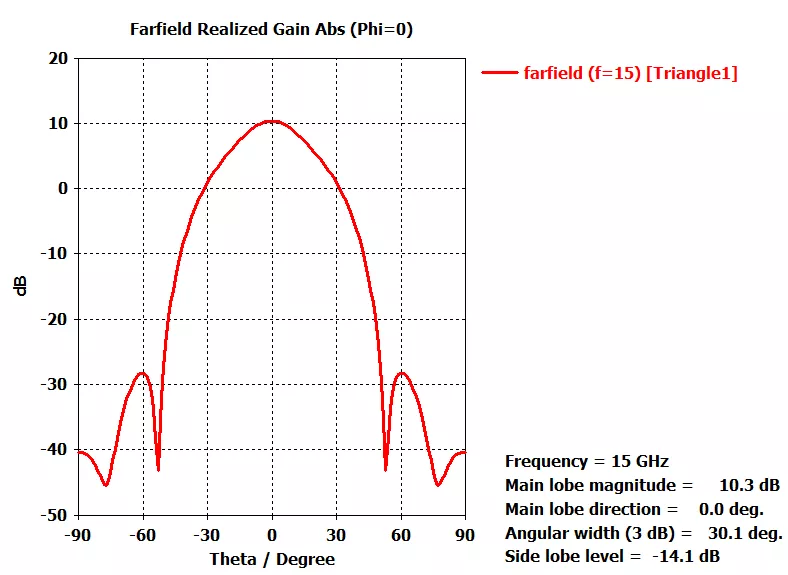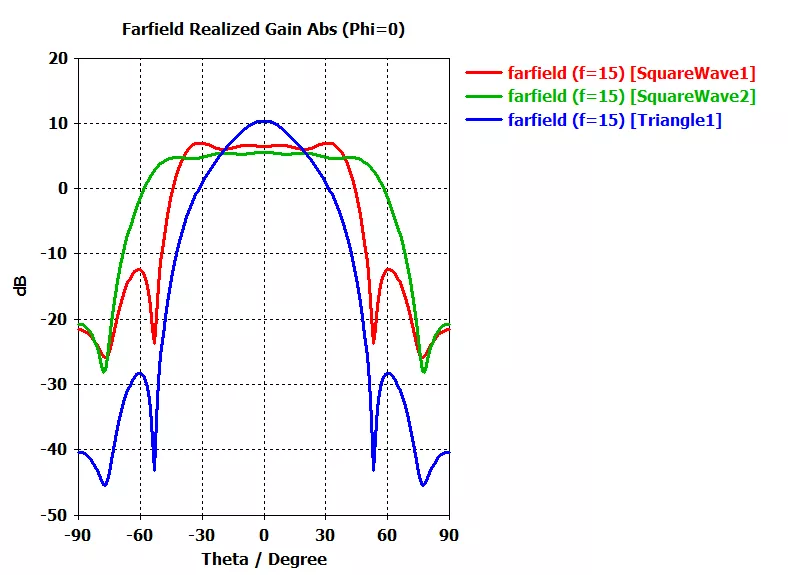END